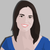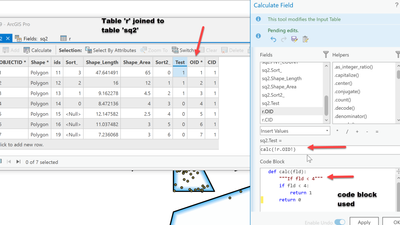# arcpy CalculateField not working as expected

560
7
12-05-2021 06:35 AMbyMVP Regular Contributor

Hello,

I am trying to use arcpy's CalculateField method on a table with a join on it. (it is my understanding that because of this, I can't use update cursors). I know the script is producing the correct results when it comes to calculating the dominant values in the list (confirmed with several print statements throughout the script) but it's just the bit at the very end that is not working the way I expected. When I run the script, only one feature gets calculated with all "Y" in the related records, and all the other features calculate with "N" in the related records.

This is the segment of the script that is giving me trouble. I'm happy to provide the full script if that would be necessary.

``````        with arcpy.da.SearchCursor(stratum_layer, PERC_COVER_FIELD) as cursor:
for row in cursor:
if int(row) in dom_list:
arcpy.management.CalculateField(stratum_layer, DOM_SPECIES_FIELD, "'Y'", "PYTHON3")
print(f"{int(row)} is dominant")
else:
arcpy.management.CalculateField(stratum_layer, DOM_SPECIES_FIELD, "'N'", "PYTHON3")
print(f"{int(row)} is not dominant")``````

This was written with Python 3.7 and executed with a script GUI in ArcGIS Pro 2.8.3

Best,
Katherine
Tags (8)
1 Solution

Accepted SolutionsbyMVP Regular Contributor

A few general remarks:

• You don't need to call perc_cover_values[sample_id]​, you already have that defined as sample_list
• You can use a where clause in SearchCursor. No need to select in the layer first.
• It's better form to let calc_veg_dom only handle the calculation and do the table manipulation in main. This way, all the input and output is in one place instead of hidden in another method.
• You don't need the sample points at all for this. You know which perc_cover values are from the same sampling site by looking at herb_stratum_repeat.parentglobalid. This also eliminates the need for CalculateField and you can use the UpdateCursor.

``````import arcpy

# GLOBAL VARIABLES
ID_FIELD = "globalid"
POINT_ID_FIELD = 'parentglobalid'
PERC_COVER_FIELD = 'abs_perc_cover_herb'
DOM_SPECIES_FIELD = 'dom_species_herb'

def calc_veg_dom(sample_dict):
"""Applies the 50/20 rule for calculating vegetation dominance. The function creates a list that identifies the
percent values that are for dominant species for a specific sampling point.

Arguments:
sample_dict: Dictionary, {ID_FIELD: PERC_COVER_FIELD}
Retuns:
Dictionary, {ID_FIELD: DOM_SPECIES_FIELD}
"""
# convert to [ [ID_FIELD, PERC_COVER_FIELD] ], so we can sort
sample_list = [[s_id, perc] for s_id, perc in sample_dict.items()]
# sort descending by PERC_COVER_FIELD
sample_list.sort(key=lambda s: s, reverse=True)
# calculate the total cover and target cover values
total_cover = sum([s for s in sample_list])
perc_cover_50 = total_cover * 0.5
perc_cover_20 = total_cover * 0.2

dom_dict = dict()
# 50% rule: species that contribute to more than 50% are dominant, species with tied cover values must be taken together
# 20% rule: species with more than 20% coverage are dominant
percentages = []  # to check for tied cover values and to calculate cover sum
for item in sample_list:
if sum(percentages) <= perc_cover_50:
percentages.append(item)
dom_dict[item] = "Y"
elif item in percentages or item >= perc_cover_20:
dom_dict[item] = "Y"
else:
dom_dict[item] = "N"

return dom_dict

###########################################################################################################

def main():
# You only need the stratum table!
fc = arcpy.GetParameterAsText(0)
stratum_table = arcpy.GetParameterAsText(1)

fields = [ID_FIELD, POINT_ID_FIELD, PERC_COVER_FIELD]
stratum_data = [row for row in arcpy.da.SearchCursor(stratum_table, fields)]

# get uniqe point_ids
point_ids = {sd for sd in stratum_data}  # set eliminates duplicates

# output dict {ID_FIELD: DOM_SPECIES_FIELD}
dom_dict = dict()

# for each point, get the perc_covers and calculate dominance
for point_id in point_ids:
# input for calc_veg_dom: {ID_FIELD: PERC_COVER_FIELD}
point_stratum_data = {sd: sd for sd in stratum_data if sd == point_id}
# output from calc_veg_dom: {ID_FIELD: DOM_SPECIES_FIELD}
point_dom_data = calc_veg_dom(point_stratum_data)
dom_dict.update(point_dom_data)

# write the results into stratum_table
fields = [ID_FIELD, DOM_SPECIES_FIELD]
with arcpy.da.UpdateCursor(stratum_table, fields) as cursor:
for s_id, dom in cursor:
try:
dom = dom_dict[s_id]
cursor.updateRow([s_id, dom])
except KeyError:
print("No dominance value found for {} = {}".format(ID_FIELD, s_id))

if __name__ == "__main__":
#main()

# test calc_veg_dom
import random
sample_dict = dict()
x = 0
while sum(sample_dict.values()) < 100:
sample_dict["id_{}".format(x)] = random.randint(5, 40)
x += 1
print("in: ", sample_dict)
print("out: ", calc_veg_dom(sample_dict))``````

Have a great day!
Johannes
7 RepliesbyMVP Esteemed Contributor

Calculate Field (Data Management)—ArcGIS Pro | Documentation

'"string"'   probably doesn't matter but ....

You can calculate with a join, just in the base table and not in the join table... sort of retired...byMVP Regular Contributor

Hi Dan,

When I said I "couldn't do it with a table" I was referring to using updateCursors instead of the CalculateField method.

The table I am trying to run the field calculation on is a many to one join.

Best,
KatherinebyMVP Esteemed Contributor

I don't see anything saying you can't use an update cursor when there are joins/relates as long as you aren't doing the calculation on the table being joined, the base table should be fine.  The field names are prepended by the table name as in my image ( table 'r' joined to table 'sq2', hence '!r.OID!'

In any event, if you want to use CalculateField, then you should be able to use the code block to do the calculation.  You don't need separate calculate field  in an if statement.

Code block and expression from my example

``````code_block = """
def calc(fld):
"""If fld < 4"""
if fld < 4:
return 1
return 0
"""``````
``"calc(!r.OID!)"``

... sort of retired...byMVP Regular Contributor

I'm using the terms of the Add Join tool: input table and join table.

Based on a quick test:

• you can not use UpdateCursor on a joined table
• doesn't matter if you try to update fields of the input table or the join table
• doesn't matter if you use the full field names or not
• you can use Calculate Field on the fields of the input table
• you have to use full names ("input_table.Field")
• you can not use Calculate Field on fields of the join table (you also can't edit them manually)

Your code doesn't work as expected, because Calculate Field calculates ALL rows, not just the one in your SearchCursor. In fact, I'm surprised that you have a feature that is different from the others.

In this case, it's probably easier to edit the table(s) on their own. Are PERC_COVER_FIELD and DOM_SPECIES_FIELD in the input table or the join table? and what are your join fields?

Have a great day!
JohannesbyMVP Regular Contributor

Hi Johannes, thanks so much for your reply! Apologies for my delayed response, I got pulled into another project this week. But I'm still hoping you can help!

PERC_COVER_FIELD and DOM_SPECIES_FIELD are both fields from the input table.

In fact, here's the full script. I'm sure that will be more helpful. Any other suggestions?

``````import arcpy

# GLOBAL VARIABLES
POINT_ID_FIELD = 'AGCP___Wetland_Determination_Data_Form_v2.sampling_point'
PERC_COVER_FIELD = 'herb_stratum_repeat.abs_perc_cover_herb'
DOM_SPECIES_FIELD = 'herb_stratum_repeat.dom_species_herb'

def unique_values(table, field):
""" Create a list that contains all the unique Sampling_Point id's in the table."""
with arcpy.da.SearchCursor(table, [field]) as cursor:
return sorted({row for row in cursor})  # using a set eliminates duplicates

def calc_veg_dom(perc_cover_values, stratum_layer):
"""Applies the 50/20 rule for calculating vegetation dominance. The function creates a list that identifies the
percent values that are for dominant species for a specific sampling point."""
for sample_id, sample_list in perc_cover_values.items():
total_cover = sum(perc_cover_values[sample_id])
perc_cover_50 = total_cover * 0.5
perc_cover_20 = total_cover * 0.2
dom_list = []

for item in sample_list:
dom_list.append(item)
# stop adding items to the list. All values that are in this list are dominant species (50% rule)
if sum(dom_list) > perc_cover_50:
break
# Identify the species that will be evaluated for the 20% rule.
# Creating a copy of the original sample_id list so we do not modify the original
remaining_vals = list(perc_cover_values[sample_id])

for item in dom_list:
if item in remaining_vals:
remaining_vals.remove(item)

for item in remaining_vals:
if item in dom_list and item < perc_cover_20:
dom_list.append(item)
if item >= perc_cover_20:
dom_list.append(item)

arcpy.management.SelectLayerByAttribute(stratum_layer, "NEW_SELECTION",
f"{POINT_ID_FIELD} = '{sample_id}'")
fields = (PERC_COVER_FIELD, DOM_SPECIES_FIELD)
exit()
with arcpy.da.SearchCursor(stratum_layer, fields) as cursor:
for row in cursor:
print(row)
if int(row) in dom_list:
arcpy.management.CalculateField(stratum_layer, DOM_SPECIES_FIELD, "'Y'", "PYTHON3")
print(f"{int(row)} is dominant")
else:
arcpy.management.CalculateField(stratum_layer, DOM_SPECIES_FIELD, "'N'", "PYTHON3")
print(f"{int(row)} is not dominant")

###########################################################################################################

def main():
fc = arcpy.GetParameterAsText(0)
stratum_table = arcpy.GetParameterAsText(1)

# Make Layer and Table View for the Join
fc_layer = arcpy.MakeTableView_management(fc, "fc_lyr")
stratum_layer = arcpy.MakeTableView_management(stratum_table, "stratum_lyr")

# Join feature class to stratum table based on the parentglobalID and globalID

point_id_list = unique_values(stratum_layer, POINT_ID_FIELD)

perc_covers_dict = {}

for id in point_id_list:
perc_covers_list = []
# Select features based on unique "Sampling_Point" name
arcpy.management.SelectLayerByAttribute(stratum_layer, "NEW_SELECTION", f"{POINT_ID_FIELD} = '{id}'")
# Create a dictionary that has all % cover numbers (value) in a list for a sampling ID (key)
with arcpy.da.SearchCursor(stratum_layer, PERC_COVER_FIELD) as cursor:
for row in cursor:
perc_covers_list.append(int(row))
perc_covers_list.sort(reverse=True)  # sort values from highest to lowest
perc_covers_dict[id] = perc_covers_list  # adds the list as the value for each key (sample id) in a dict

calc_veg_dom(perc_covers_dict, stratum_layer)

if __name__ == "__main__":
main()
``````

Best,
KatherinebyMVP Regular Contributor

A few general remarks:

• You don't need to call perc_cover_values[sample_id]​, you already have that defined as sample_list
• You can use a where clause in SearchCursor. No need to select in the layer first.
• It's better form to let calc_veg_dom only handle the calculation and do the table manipulation in main. This way, all the input and output is in one place instead of hidden in another method.
• You don't need the sample points at all for this. You know which perc_cover values are from the same sampling site by looking at herb_stratum_repeat.parentglobalid. This also eliminates the need for CalculateField and you can use the UpdateCursor.

``````import arcpy

# GLOBAL VARIABLES
ID_FIELD = "globalid"
POINT_ID_FIELD = 'parentglobalid'
PERC_COVER_FIELD = 'abs_perc_cover_herb'
DOM_SPECIES_FIELD = 'dom_species_herb'

def calc_veg_dom(sample_dict):
"""Applies the 50/20 rule for calculating vegetation dominance. The function creates a list that identifies the
percent values that are for dominant species for a specific sampling point.

Arguments:
sample_dict: Dictionary, {ID_FIELD: PERC_COVER_FIELD}
Retuns:
Dictionary, {ID_FIELD: DOM_SPECIES_FIELD}
"""
# convert to [ [ID_FIELD, PERC_COVER_FIELD] ], so we can sort
sample_list = [[s_id, perc] for s_id, perc in sample_dict.items()]
# sort descending by PERC_COVER_FIELD
sample_list.sort(key=lambda s: s, reverse=True)
# calculate the total cover and target cover values
total_cover = sum([s for s in sample_list])
perc_cover_50 = total_cover * 0.5
perc_cover_20 = total_cover * 0.2

dom_dict = dict()
# 50% rule: species that contribute to more than 50% are dominant, species with tied cover values must be taken together
# 20% rule: species with more than 20% coverage are dominant
percentages = []  # to check for tied cover values and to calculate cover sum
for item in sample_list:
if sum(percentages) <= perc_cover_50:
percentages.append(item)
dom_dict[item] = "Y"
elif item in percentages or item >= perc_cover_20:
dom_dict[item] = "Y"
else:
dom_dict[item] = "N"

return dom_dict

###########################################################################################################

def main():
# You only need the stratum table!
fc = arcpy.GetParameterAsText(0)
stratum_table = arcpy.GetParameterAsText(1)

fields = [ID_FIELD, POINT_ID_FIELD, PERC_COVER_FIELD]
stratum_data = [row for row in arcpy.da.SearchCursor(stratum_table, fields)]

# get uniqe point_ids
point_ids = {sd for sd in stratum_data}  # set eliminates duplicates

# output dict {ID_FIELD: DOM_SPECIES_FIELD}
dom_dict = dict()

# for each point, get the perc_covers and calculate dominance
for point_id in point_ids:
# input for calc_veg_dom: {ID_FIELD: PERC_COVER_FIELD}
point_stratum_data = {sd: sd for sd in stratum_data if sd == point_id}
# output from calc_veg_dom: {ID_FIELD: DOM_SPECIES_FIELD}
point_dom_data = calc_veg_dom(point_stratum_data)
dom_dict.update(point_dom_data)

# write the results into stratum_table
fields = [ID_FIELD, DOM_SPECIES_FIELD]
with arcpy.da.UpdateCursor(stratum_table, fields) as cursor:
for s_id, dom in cursor:
try:
dom = dom_dict[s_id]
cursor.updateRow([s_id, dom])
except KeyError:
print("No dominance value found for {} = {}".format(ID_FIELD, s_id))

if __name__ == "__main__":
#main()

# test calc_veg_dom
import random
sample_dict = dict()
x = 0
while sum(sample_dict.values()) < 100:
sample_dict["id_{}".format(x)] = random.randint(5, 40)
x += 1
print("in: ", sample_dict)
print("out: ", calc_veg_dom(sample_dict))``````

Have a great day!
JohannesbyMVP Regular Contributor

Johannes,

You have gone above and beyond in assisting me with this. Not only does your solution work, it is more elegant and really helps a novice like me see how I can clean up my coding style. I can't thank you enough!

"Give a Man a Fish, and You Feed Him for a Day. Teach a Man To Fish, and You Feed Him for a Lifetime."

Best,

Katherine

Best,
Katherine